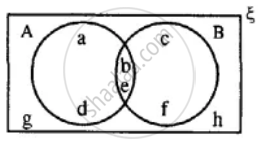# Using the Given Diagram, Express the Following Sets in the Terms of a and B. - Mathematics

Diagram
Sum

Using the given diagram, express the following sets in the terms of A and B. {a, d, g, h}#### Solution

{a, d, g, h} = B'

[∵ {b, c, e, f} = B ∴ B' = {a, d, g, h}]

Concept: Venn Diagram
Is there an error in this question or solution?

#### APPEARS IN

Selina Concise Mathematics Class 8 ICSE
Chapter 6 Sets
Exercise 6 (E) | Q 11.4 | Page 76